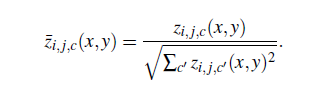# Specialized Normalization Running Slow in Pytorch

I have a tensor of shape `z = (38, 38, 7, 7, 21) = (x_pos, y_pos, grid_i, grid_j, class_num)`, and I wish to normalize it according to the formula:I have produced a working example of what I mean here, and the problem is that it is extremely slow, approximately 2-3 seconds for each grid entry (of which there are 49, so 49*3 seconds = 147 seconds, which is way too long, considering I need to do this with thousands of image feature maps).
Any optimizations or obvious problems very much appreciated. This is part of a Pytorch convolutional neural network architecture, so I am using torch tensors and tensor ops.

``````import torch
def normalizeScoreMap(score_map):
for grid_i in range(7):
for grid_j in range(7):
for x in range(38):
for y in range(38):
grid_sum = torch.tensor(0.0).cuda()
for class_num in range(21):
grid_sum += torch.pow(score_map[x][y][grid_i][grid_j][class_num], 2)
grid_normalizer = torch.sqrt(grid_sum)
for class_num in range(21):
score_map[x][y][grid_i][grid_j][class_num] /= grid_normalizer
return score_map

random_score_map = torch.rand(38,38,7,7,21).cuda()
score_map = normalizeScoreMap(random_score_map)
``````

Edit: For reference I have an i9-9900K CPU and a nvidia 2080 GPU, so my hardware is quite good. I would be willing to try multi-threading but I am looking for more obvious problems/optimizations.

This should work:

``````x = random_score_map.clone()
s = (x**2).sum(4, keepdims=True)
n = torch.sqrt(s)
x /= n

print(torch.allclose(x, score_map))
> True
``````

Note that you should avoid for loops where possible and try to use vectorized code instead.